# NCERT Solutions for Class 8 Maths Chapter 10 Visualizing Solid Shapes

NCERT solutions for class 8 maths chapter 10 Visualizing Solid Shapes- You have already learnt about the 2D geometry in which basic shapes like a circle, a rectangle, square or rhombus are measured by length and width. We live in a 3-Dimensional world where every object that we can see or touch has three dimensions length, width, and height. For example, the room can be described by 3-dimensions length, width, and height. In this article, you will get NCERT solutions for class 8 maths chapter 10 visualizing solid shapes that are prepared and explained in a detailed manner to clear your doubts. In this chapter, you will study solid objects like cubes, cuboids, cones, spheres, hemispheres, etc. You must have noticed that 3-dimensional objects look different when you look from different positions or different angles. To simplify its study there are defined viewpoints (front view, side view, top view) for the 3D object.

In solutions of NCERT for class 8 maths chapter 10 visualizing solid shapes, maximum questions are related to finding front view, side view, and top view of the 3D geometry. Important topics like views of 3D shapes, mapping space around us, faces, edges, and vertices of the objects are covered in this chapter. CBSE NCERT solutions for class 8 maths chapter 10 visualizing solid shapes are very helpful in engineering drawing, locating the place and will build a basic understanding of the 3D shapes. You can find NCERT solutions from class 6 to 12 by clicking on the above link.

Let's understand this chapter with the help of an example of a brick with its front view, side view, top view.You can visualize a few more shapes your self.

For example: Take a cylinder. What is the side view of it? Is circular? Now take bangles of the same size and hold them together. Is it looks like a cylinder?

from the above-mentioned activity, we observed that when we hold a few bangles which are circular in shape with small thicknesses together we obtained a hollow cylinder.

## Important topics of NCERT Class 8 Maths Chapter 10 Visualizing Solid Shapes-

• 10.1 Introduction
• 10.2 Views of 3D-Shapes
• 10.3 Mapping Space Around Us
• 10.4 Faces, Edges, and Vertices

## NCERT solutions for class 8 maths chapter 10 visualizing solid shapes topic 10.1 introduction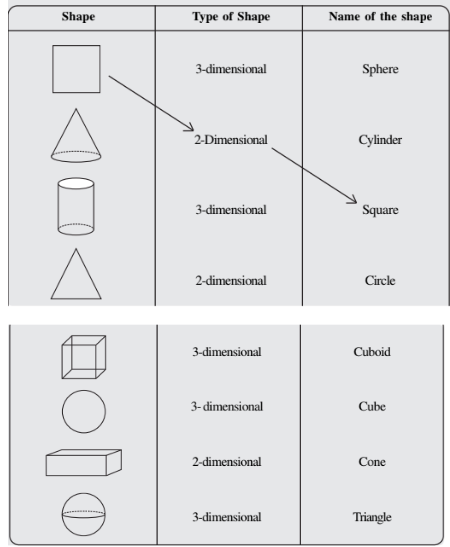Matched items are: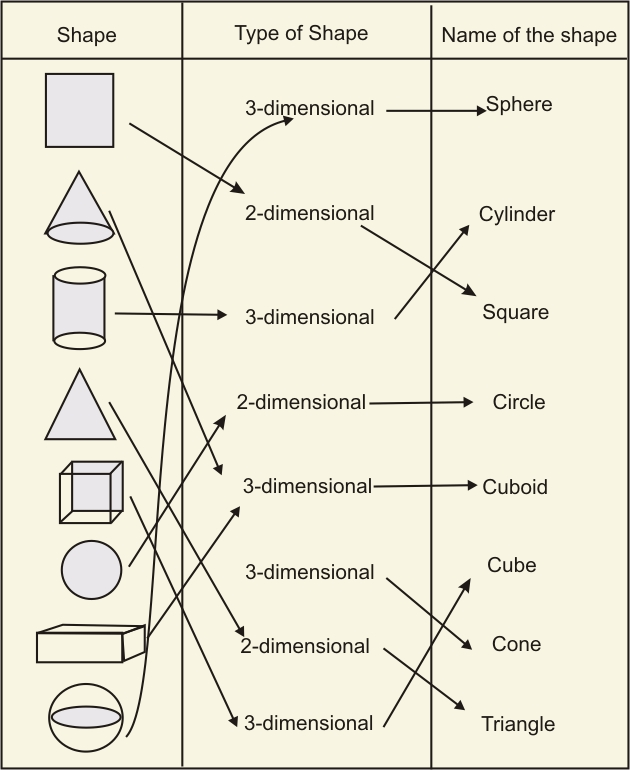Picture (object)

Shape

(i) An agricultural fieldTwo rectangular cross paths inside a rectangular park.

(ii) A grooveA circular path around a circular ground.

(iii) A toyA triangular field adjoining a square field.

(iv) A circular parkA cone taken out of a cylinder.

(v) A cross pathA hemisphere surmounted on a cone.

Matched objects are:Solutions of NCERT for class 8 chapter 10 visualizing solid shapes-Exercise: 10.1

Object                                Side view                                        Top viewMatched objects are:

(1) →iii)→iv)

(2) →i) →v)

(3) →iv)→ii)

(4) →v) →iii)

(5) →ii) →i)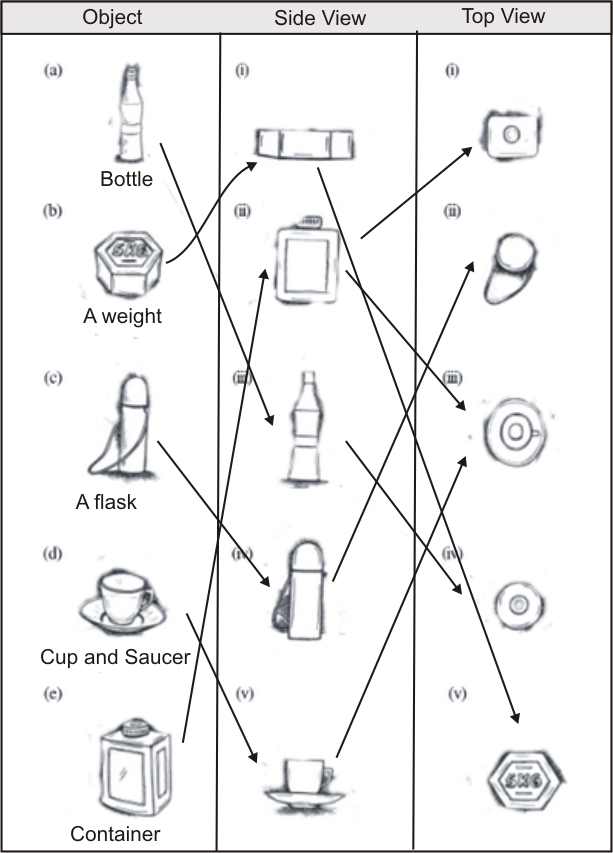(a) Different views have given:  (i) Front, (ii) Side, (iii) Top.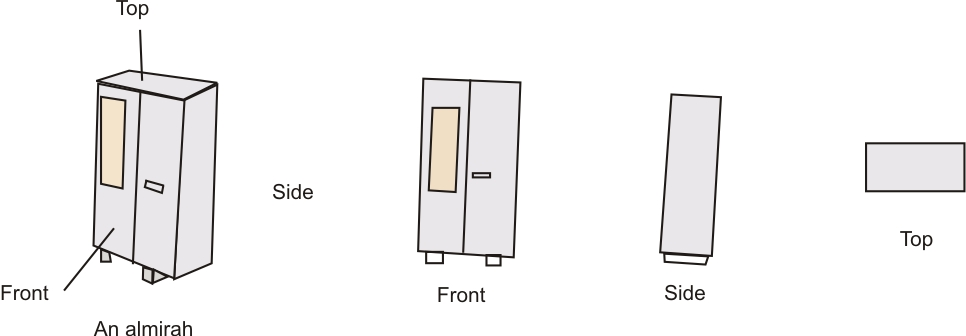(b)  (i) Side view, (ii) Front view, (iii) Top view.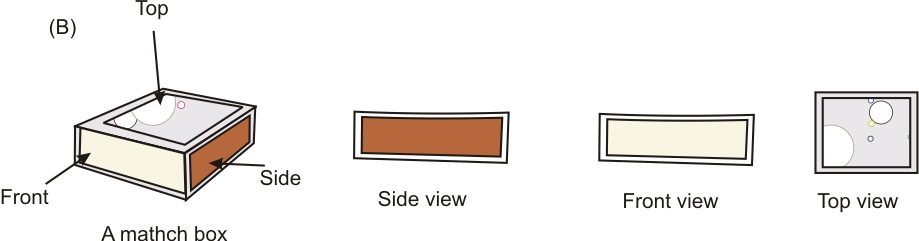(c) (i) Front view, (ii) Side view, and (iii) Top view.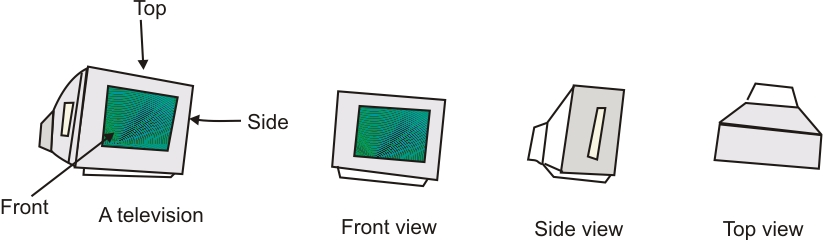(d) (i) Front view, (ii) Side view, and (iii) Top view.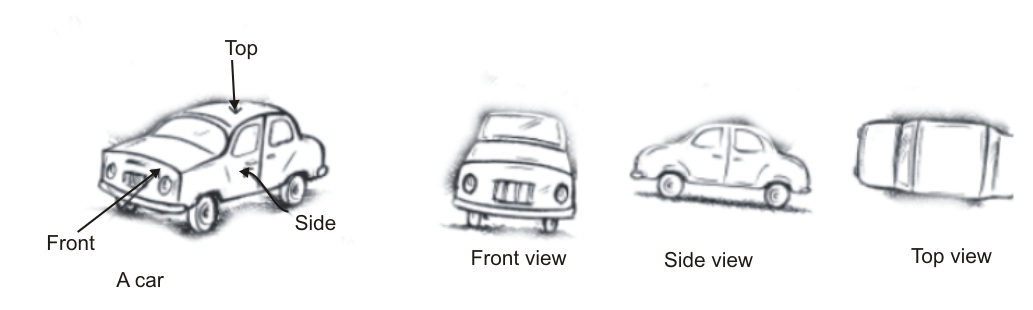(a) (i) Top view, (ii) Front/ Side view, and (iii) Side/ Front view.(b) (i) Side view, (ii) Front view, and (iii) Top view.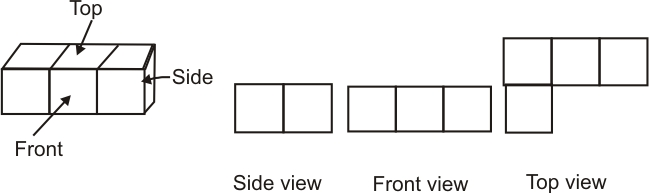(c) (i) Top view, (ii) Side view, and (iii) Front view.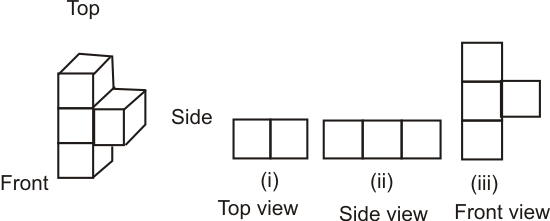(d) (i) Side view, (ii) Front view, and (iii) Top view.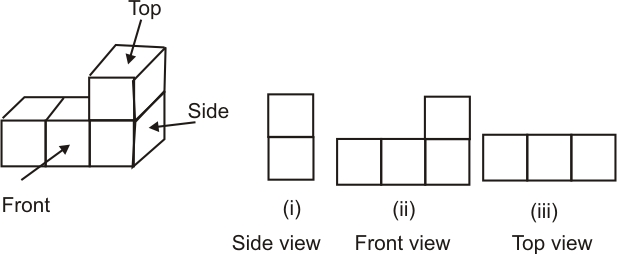(e) (i) Front view, (ii) Top view, and (iii) Side view.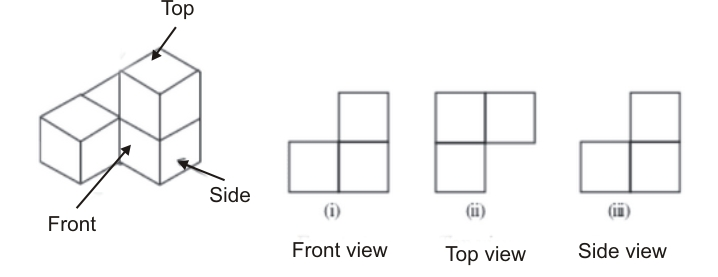A military tentThe front view, top view, and side view of a tent are shown below:A tableFront view, Top view, and Side view of the table shown below:A nutThe front, top, and side views of the nut are: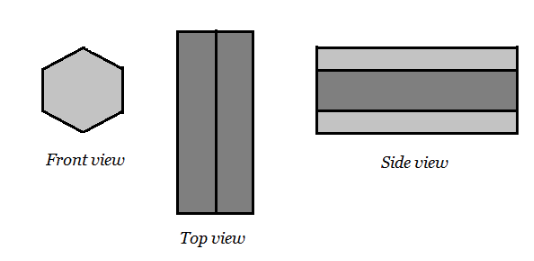A hexagonal blockThe front view, side view and top view of the hexagonal block are shown below:The front view, side view and top view of the dice as shown below:A solidThe front view, side view and top view of the given solid.CBSE NCERT solutions for class 8 maths chapter 10 visualizing solid shapes-Exercise: 10.2

Question:1(a) Look at the given map of a city.Colour the map as follows: Blue-water, red-fire station, orange-library, yellow
schools, Green - park, Pink - College, Purple - Hospital, Brown - Cemetery.

(a) Coloured map shown:Do it yourself.

Do it with your own imagination.

Do it with your own imagination.

NCERT solutions for class 8 maths chapter 10 visualizing solid shapes topic 10.4 faces, edges and vertices

Solid

F

V

E

F+V

E+2

Cuboid

Triangular pyramid

Triangular prism

Pyramid with square base

Prism with square base

Solid

F

V

E

F+V

E+2

Cuboid

6

8

12

14

14

Triangular pyramid

4

4

6

8

8

Triangular prism

5

6

9

11

11

Pyramid with square base

5

5

8

10

10

Prism with square base

6

8

12

14

14

## Solutions of NCERT for class 8 maths chapter 10 visualizing solid shapes-Exercise: 10.3

Question:1(i) Can a polyhedron have for its faces

3 triangles?

(i) No, a polyhedron cannot have 3 triangles for its faces

Question:1(ii) Can a polyhedron have for its faces

4 triangles?

(ii) Yes, a polyhedron having 4 triangles is pyramid on triangular base.

Question:1(iii) Can a polyhedron have for its faces

a square and four triangles?

(iii) Yes, a polyhedron can have faces of a square and four triangles which makes a pyramid on square base.

Yes, it is possible if the number of faces is four or more than four.

Question:3(i) Which are prisms among the following?(i) a nail is not a prism.

Question:3(ii) Which are prisms among the following?(ii) The unsharpened pencil is a prism.

Question:3(iii) Which are prisms among the following?(iii) Table weight is not a prism.

Question:3(iv) Which are prisms among the following?(iv) Given box is a prism.

Question:4(i) How are prisms and cylinders alike?

(i) A prism is a polyhedron whose base and top are congruent polygons and whose other faces, i.e., lateral faces are parallelograms in shape. Also, the cylinder has base on top and bottom and faces are parallel.

No, not always, because it can be a cuboid also when extended.

Question:6(i) Verify Euler’s formula for these solids.(i) Euler formula F+V = E + 2.

So, Number of faces (F) = 7, Number of vertices (V)  = 10 and number of edges (E)  = 15.

Hence putting in the formula; we get,

7 + 10 = 15 + 2

17 = 17 hence, it satisfies the Euler formula.

Question:6(ii) Verify Euler’s formula for these solids.(ii) Euler formula F+V = E + 2.

So, Number of faces (F) = 9, Number of vertices (V)  = 9 and number of edges (E)  = 16.

Hence putting in the formula; we get,

9 + 9 = 16 + 2

18 = 18 hence, it satisfies the Euler formula.

Question:7 Using Euler’s formula find the unknown.We know the Euler Formula:

F + V = E + 2:

hence we can easily find the unknown let say 'F, V, and E'

So, For Ist case:

F + 6  = 12 + 2  or  F = 8, hence the number of faces in Ist case is 8.

For IInd Case;

5 + V = 9 + 2   or  V = 6, hence the number of vertices in IInd case is 6.

For IIIrd case;

20 + 12 = E + 2     or   E = 30, hence the number of Edges in the IIIrd case is 30.

 Faces 8 5 20 Vertices 6 6 12 Edges 12 9 30

Checking using Euler's Formula:

F + V = E +2;

Given that F = 10, V = 15 and E = 20 so,

Putting in equation: 10 + 15 = 35  and 20 +2 = 22

Hence, given polyhedron having 10 faces, 20 edges and 15 vertices is not possible.

## NCERT Solutions For Class 8 Maths: Chapter-wise

 Chapter -1 NCERT solutions for class 8 maths chapter 1 Rational Numbers Chapter -2 Solutions of NCERT for class 8 maths chapter 2 Linear Equations in One Variable Chapter-3 CBSE NCERT solutions for class 8 maths chapter 3 Understanding Quadrilaterals Chapter-4 NCERT solutions for class 8 maths chapter 4 Practical Geometry Chapter-5 Solutions of NCERT for class 8 maths chapter 5 Data Handling Chapter-6 CBSE NCERT solutions for class 8 maths chapter 6 Squares and Square Roots Chapter-7 NCERT solutions for class 8 maths chapter 7 Cubes and Cube Roots Chapter-8 Solutions of NCERT for class 8 maths chapter 8 Comparing Quantities Chapter-9 NCERT solutions for class 8 maths chapter 9 Algebraic Expressions and Identities Chapter-10 CBSE NCERT solutions for class 8 maths chapter 10 Visualizing Solid Shapes Chapter-11 NCERT solutions for class 8 maths chapter 11 Mensuration Chapter-12 Solutions of NCERT for class 8 maths chapter 12 Exponents and Powers Chapter-13 CBSE NCERT solutions for class 8 maths chapter 13 Direct and Inverse Proportions Chapter-14 NCERT solutions for class 8 maths chapter 14 Factorization Chapter-15 Solutions of NCERT for class 8 maths chapter 15 Introduction to Graphs Chapter-16 CBSE NCERT solutions for class 8 maths chapter 16 Playing with Numbers

## NCERT Solutions For Class 8: Subject-Wise

In CBSE NCERT solutions for class 8 maths chapter 10 visualizing solid shapes only one formula has been used know as Euler’s formula.

For any polyhedron-

where F - Number of faces

V - Number of vertices

E - Number of edges

As the name suggests this chapter is more about visualizing the shapes and drawing the front view, side view, and top view. In exercise 1 and 2 of NCERT solutions for class 8 maths chapter 10 visualizing solid shapes, most questions are related to visualizing shape and mapping which are explained with the help of drawing and graphs.